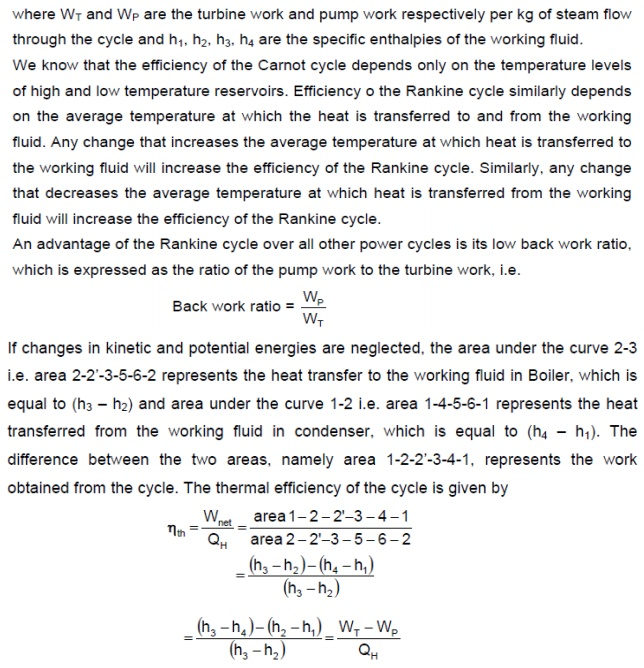Home | | Engineering Thermodynamics | Gas Power / Air Standard Cycles

# Gas Power / Air Standard Cycles

For small power plants, gas is ideally preferred as the working fluid. The gasoline engines, diesel engines and gas turbines are common examples.

GAS POWER / AIR STANDARD CYCLES.

For small power plants, gas is ideally preferred as the working fluid. The gasoline engines, diesel engines and gas turbines are common examples. The analysis of the air-standard cycle is based on the assumptions that are far from real. In actual internal combustion (IC) engines, chemical reaction occurs inside the engine cylinder as a result of combustion of air-fuel mixture and. The IC engines are actually operated on Open cycles in which the working fluid does not go through a cycle. The accurate analysis of IC engine is very complicated. However, it is advantageous to analyse the performance of an ideal closed cycle that closely approximates the real cycle. One such approach is air-standard cycle, which is based on certain assumptions. The assumptions for idealized air-standard cycles are:

1)   The working fluid, air, is assumed to be an ideal gas. The equation of state is given by the equation pv = RT and the specific heats are assumed to be constant.

2)  All processes that constitute the cycle are reversible.

3)    No chemical reaction occurs during the cycle. Heat is supplied from a high temperature reservoir (instead of chemical reaction) and some heat is rejected to the low temperature reservoir during the cycle.

4)  The mass of air within the system remains constant throughout the cycle.

5) Heat losses from the system to the atmosphere are assumed to be zero. In this we shall discuss about the Brayton cycle, Otto cycle and Diesel cycle.

BRAYTON CYCLE

The Brayton cycle is widely used as the basis for the operation of Gas turbine. A schematic diagram of a simple gas turbine (open cycle) and the corresponding p-v and T-s diagrams are shown below. Air is drawn from he atmosphere into compressor, where it is compressed reversibly and adiabatically. The relative high pressure air is then used in burning the fuel in the combustion chamber. The air-fuel ratio quite high (about 60:1) to limit the temperature burnt gases entering the turbine. The gases then expand isentropically in the turbine. A portion of the work obtained from the turbine is utilised to drive the compressor and the auxiliary drive and the rest of the power output is the net power of the gas turbine plant.Simple gas turbine Brayton cycle with closed cycle consists ofRANKINE CYCLECOMBINED CYCLEThermal Efficiency is given as above.OTTO CYCLE

The air standard Otto Cycle is an idealised cycle which closely approximates the operation of Spark Ignition (SI) Engine.Process 1-2 is reversible adiabatic (isentropic) compression of air when the piston moves from crank-end dead centre (BDC) to cylinder head dead centre (TDC). During the process 2-3, heat is transferred reversibly to the system at constant volume (this process corresponds to the spark ignition of the actual engine). The curve 3-4 represents the reversible adiabatic expansion process when piston moves from TDC to BDC and the curve 4-1 represents the heat rejected by the system reversibly at constant volume.Study Material, Lecturing Notes, Assignment, Reference, Wiki description explanation, brief detail
Mechanical : Engineering Thermodynamics : Properties of a Pure Substance and Steam Power Cycle : Gas Power / Air Standard Cycles |